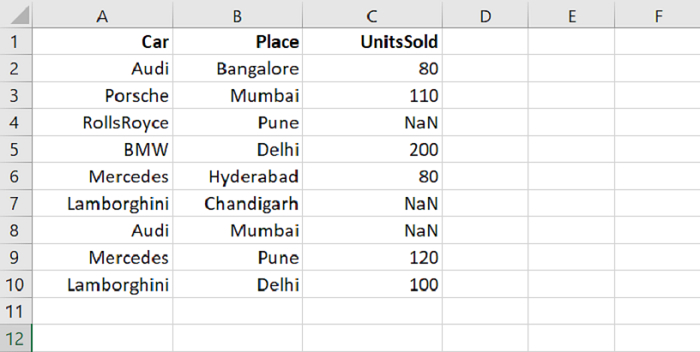# Python Pandas – Check for Null values using notnull()

PythonServer Side ProgrammingProgramming

The notnull() method returns a Boolean value i.e. if the DataFrame is having null value(s), then False is returned, else True.

Let’s say the following is our CSV file with some NaN i.e. null values −Let us first read the CSV file −

dataFrame = pd.read_csv("C:\\Users\\amit_\\Desktop\\CarRecords.csv")

Checking for not null values −

res = dataFrame.notnull()

Now, on displaying the DataFrame, the CSV data will be displayed in the form of True and False i.e. boolean values because notnull() returns boolean. For Null values, False will get displayed. For Not-Null values, True will get displayed.

## Example

Following is the complete code −

import pandas as pd

print("DataFrame...\n",dataFrame)

# count the rows and columns in a DataFrame
print("\nNumber of rows and column in our DataFrame = ",dataFrame.shape)

res = dataFrame.notnull()
print("\nDataFrame displaying False for Null (NaN) value = \n",res)

dataFrame = dataFrame.dropna()
print("\nDataFrame after removing null values...\n",dataFrame)
print("\n(Updated) Number of rows and column in our DataFrame = ",dataFrame.shape)

## Output

This will produce the following output −

DataFrame...
Car       Place   UnitsSold
0         Audi   Bangalore        80.0
1      Porsche      Mumbai       110.0
2   RollsRoyce        Pune         NaN
3          BMW       Delhi       200.0
5  Lamborghini  Chandigarh         NaN
6         Audi      Mumbai         NaN
7     Mercedes        Pune       120.0
8  Lamborghini       Delhi       100.0

Number of rows and column in our DataFrame = (9, 3)

DataFrame displaying False for Null values =
Car   Place   UnitsSold
0  True    True        True
1  True    True        True
2  True    True       False
3  True    True        True
4  True    True        True
5  True    True       False
6  True    True       False
7  True    True        True
8  True    True        True

DataFrame after removing null values...
Car      Place   UnitsSold
0         Audi  Bangalore        80.0
1      Porsche     Mumbai       110.0
3          BMW      Delhi       200.0
(Updated)Number of rows and column in our DataFrame = (6, 3)